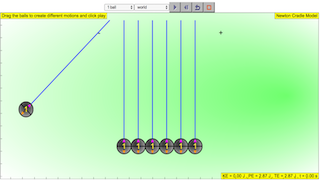Intro Page

<p>Newton's cradle is a device that demonstrates conservation of momentum and energy using a series of swinging spheres. When one sphere at the end is lifted and released, it strikes the stationary spheres, transmitting a force through the stationary spheres that pushes the last sphere upward. The last sphere swings back and strikes the still nearly stationary spheres, repeating the effect in the opposite direction. The device is named after 17th-century English scientist Sir Isaac Newton. It is also known as Newton's pendulum, Newton's balls, Newton's rocker or executive ball clicker (since the device makes a click each time the balls collide, which they do repeatedly in a steady rhythm).</p>

<p>A typical Newton's cradle consists of a series of identically sized metal balls suspended in a metal frame so that they are just touching each other at rest. Each ball is attached to the frame by two wires of equal length angled away from each other. This restricts the pendulums' movements to the same plane.</p>

<h2>Controls</h2>

<p>The first comboBox allows eay selection of number of balls in the Newton's cradle</p>

<p> 1 ball to the left</p>

<p> 2 balls to the left</p>

<p> 3 balls to the left</p>

<p> 4 balls to the left</p>

<p> 5 balls to the left</p>

<p> 6 balls to the left</p>

<p> 7 balls to the left</p>

<p> 1 ball 1 raises 1 ball to the left and 1 ball to the right</p>

<p> 2 ball 1 raises 2 ball to the left and 1 ball to the right</p>

<p> 2 ball 2 raises 2 balls to the left and 2 balls to the right</p>

<p> 3 ball 3 raises 3 balls to the left and 3 balls to the right</p>

<p>n is the number of balls allowed</p>

<p>i is the index of the ball to change</p>

<p>for which m[i] is the mass of the index ball</p>

<p>play button starts the simulation</p>

<p>reset button starts the simulation at afresh</p>

<h2>Physics explanation</h2>

<p>Newton's cradle can be modeled fairly accurately with simple mathematical equations with the assumption that the balls always collide in pairs.</p>

<p> The motion of the balls are modelled using $$\frac{d\theta[i]}{dt} = \omega[i]$$ </p>

<p>where $$\theta$$ is the angle of the ball</p>

<p> $$\omega$$ is the angular velocity </p>

<p> i represents each element of the ball</p>

<p> t is time </p>

<p> the second order differential equation is $$\frac{d(\omega[i])}{dt}=-\frac{g*sin(\theta[i])}{L}-k*L*\omega[i]$$ </p>

<p> where g is gravitational constant 9.81 $$m/s^2$$ </p>

<p> L is the length of the inextensible string connecting the ball to the support for rotational motion </p>

<p> k is a resistant coefficient to rotational motion $$\omega$$ </p>

<h2>Events of the ordinary differential equations</h2>

<p>In a state event, a TOLERANCE =1.0e-6 is defined, a loop to check through each ball i, where a check of the $$\theta[i]$$ is greater than $$\theta[i+1]$$ plus the TOLERANCE, if true, return the balls to their closed up angular position $$\theta[i+1]-\theta[i]$$. If false, do nothing. </p>

<p>The following actions are carried out at the end of the event</p>

<p>$$m_{1}=m[cid]$$ where $$m_{1}$$ is a dummy variable to contain mass of collision with index = cid</p>

<p>$$m_{2}=m[cid+1]$$;where $$m_{2}$$ is a dummy variable to contain mass of collision with index = cid+1</p>

<p>$$v_{1}=L*\omega[cid]$$;</p>

<p>$$v_{2}=L*\omega[cid+1]$$;</p>

<p>$$v_{a}=\frac{((m_{1}-m_{2})*v_{1}+2*m_{2}*v_{2})}{(m_{1}+m_{2})}$$;// velocity after collision</p>

<p>$$v_{b}=\frac{(2*m_{1}*v_{1}+(m_{2}-m_{1})*v_{2})}{(m_{1}+m_{2})}$$;</p>

<p>$$\omega[cid]=\frac{v_{a}}{L}$$;// back to omega</p>

<p>$$\omega[cid+1]=\frac{v_{b}}{L}$$;</p>

<h2>Fixed Relationship Calculations</h2>

<p>There is a for loop over i=0 to i less than number of balls to calculate the following to constraint the masses to move in pendulum-part of a circular path</p>

<p> $$dx[i]=L*(sin \theta[i])$$ where dx[i] is the change is x[i] position</p>

<p> $$dy[i]=-L*cos(\theta[i])$$ where dy[i] is the change in y[i] position</p>

<p> x[i]=xc[i]+dx[i] where xc[i] is each balls centre or equilibrium x position</p>

<p> y[i]=ymax+dy[i] where ymax is the support y position</p>

<p> $$vx[i]=L*\omega[i]*cos(\theta[i])$$ where vx[i] is the x direction linear velocities of each ball</p>

<p> $$vy[i]=L*\omega[i]*sin(\theta[i])$$ where vy[i] is the y direction linear velocities of each ball</p>

### Translations

Code Language Translator Run### Software Requirements

SoftwareRequirements

 Android iOS Windows MacOS with best with Chrome Chrome Chrome Chrome support full-screen? Yes. Chrome/Opera No. Firefox/ Samsung Internet Not yet Yes Yes cannot work on some mobile browser that don't understand JavaScript such as..... cannot work on Internet Explorer 9 and below

### CreditsFu-Kwun Hwang and lookang (This email address is being protected from spambots. You need JavaScript enabled to view it.); lookang; Francisco Esquembre

### Other Resources

2. See the Pen Newton's Cradle by liabru (@liabru) on CodePen.

# Testimonials (0)

There are no testimonials available for viewing. Login to deploy the article and be the first to submit your review!